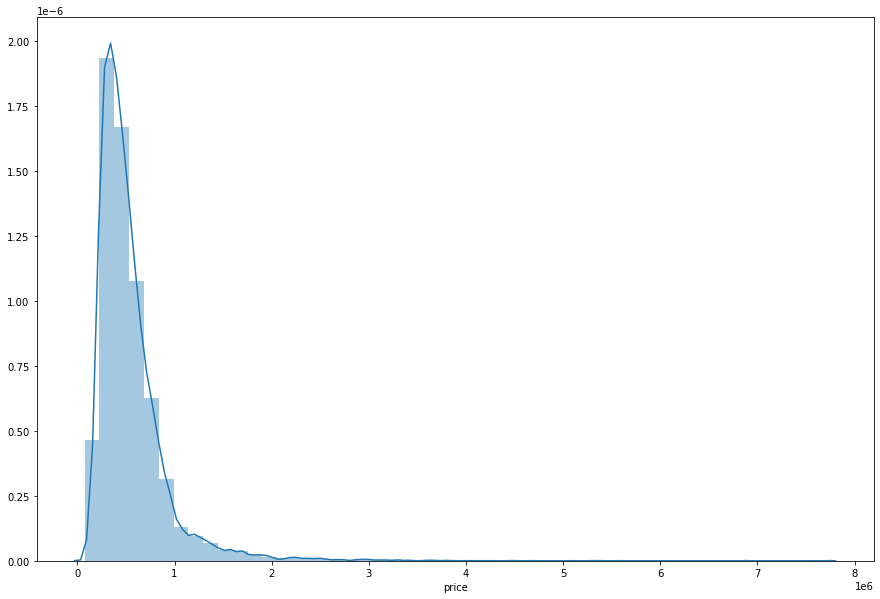Search

# Multivariable Linear Regression: A practical approach with python

In my previous blog, you can find out what is Linear Regression and how many types of Linear Regression. Click here to read the blog. We will see How to implement Multivariable linear regression. Now we take a simple dataset to find the linear regression between multi variables. You can find out the dataset on the given link.This is the dataset where different variables represent the different parameters that might affect the prediction of house pricing.

We will first load the dataset in python using panda and then we will plot the data to scatter plot. Then we will apply variables to X and Y-axis. Then we will Import the Linear Regression model from scikit learn. After that, we will find the predicted and an error value. The final step is to find the intercept and coefficient of the line.

```import pandas as pd
import numpy as np
import matplotlib.pyplot as plt
import seaborn as seabornInstance
from sklearn.model_selection import train_test_split
from sklearn.linear_model import LinearRegression
from sklearn import metrics
%matplotlib inline```

```dataset = pd.read_csv('../input/housesalesprediction/kc_house_data.csv')
dataset```

Now we will find the null value in each column.

`dataset.isnull().any()`

If any null column is there we will fill that column or cell using ffill method.

`dataset = dataset.fillna(method='ffill')`

Now we will give the variables to the X and Y-axis.

```X = dataset[['bedrooms', 'bathrooms', 'sqft_living', 'sqft_lot', 'floors', 'waterfront', 'view', 'condition', 'grade', 'sqft_above','sqft_basement','yr_built','yr_renovated','sqft_living15','sqft_lot15']].values
y = dataset['price'].values```

Here, if we use the scatter plot with multiple data we will have multiple graphs.

So, here we will be visualizing the distribution of the dataset using distplot.

```plt.figure(figsize=(15,10))
plt.tight_layout()
seabornInstance.distplot(dataset['price'])```Now we will train the dataset.

`X_train, X_test, y_train, y_test = train_test_split(X, y, test_size=0.2, random_state=0)`

Apply Linear regression to the training dataset.

```regressor = LinearRegression()
regressor.fit(X_train, y_train)```

Output: LinearRegression()

In this trained dataset, we will find the predicted values of the price that is Y-axis.

```y_pred = regressor.predict(X_test)
df = pd.DataFrame({'Actual': y_test, 'Predicted': y_pred})
print(df1)```

Output :

Actual Predicted 0 297000.0 2.921765e+05 1 1578000.0 1.494760e+06 2 562100.0 5.066236e+05 3 631500.0 5.628136e+05 4 780000.0 8.488222e+05 5 485000.0 2.965834e+05 6 340000.0 4.558610e+05 7 335606.0 5.471087e+05 8 425000.0 6.467939e+05 9 490000.0 1.180453e+06 10 732000.0 6.828250e+05 11 389700.0 2.804594e+05 12 450000.0 3.083972e+05 13 357000.0 3.457108e+05 14 960000.0 8.476072e+05 15 257000.0 4.324781e+05 16 448000.0 2.629458e+05 17 610000.0 6.340113e+05 18 230950.0 3.254760e+05 19 377500.0 4.836650e+05 20 375000.0 3.630219e+05 21 410000.0 4.253476e+05 22 459000.0 5.174309e+05 23 190000.0 2.323108e+05 24 585000.0 5.819108e+05

Now we will plot the bar graph of actual value vs predicted value.

```df1.plot(kind='bar',figsize=(10,8))
plt.grid(which='major', linestyle='-', linewidth='0.5', color='green')
plt.grid(which='minor', linestyle=':', linewidth='0.5', color='black')
plt.show()```Here we will find the error to find out the difference between what the model is predicting and the actual value.

```print('Mean Absolute Error:', metrics.mean_absolute_error(y_test, y_pred))
print('Mean Squared Error:', metrics.mean_squared_error(y_test, y_pred))
print('Root Mean Squared Error:', np.sqrt(metrics.mean_squared_error(y_test, y_pred)))```

Output:

Mean Absolute Error: 135845.78030675807

Mean Squared Error: 42149578504.03694

Root Mean Squared Error: 205303.62516048504

See All